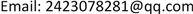﻿ 基于Logistic回归模型的高维数据变量选择方法比较研究 A Comparative Study of Variable Selection Methods for High Dimensional Data Based on Logistic Regression Model

Statistics and Application
Vol. 08  No. 03 ( 2019 ), Article ID: 31078 , 7 pages
10.12677/SA.2019.83062

A Comparative Study of Variable Selection Methods for High Dimensional Data Based on Logistic Regression Model

Dan Liao

College of Science, North China University of Technology, BeijingReceived: Jun. 6th, 2019; accepted: Jun. 21st, 2019; published: Jun. 28th, 2019ABSTRACT

High-dimensional data has become a hot research field in modern large data analysis. Variable selection is a widely-used method for high-dimensional data analysis. A large number of high-dimensional variable selection methods have appeared in the literatures. In order to compare the scope of application, advantages and disadvantages of several influential methods, in this paper, we consider the variable selection methods such as lasso and adaptive lasso to study the variable selection problem in logistic regression model. Firstly, by random simulation experiments, we compare the prediction and selection effects of different variable selection methods in low and high dimensions respectively. Then, we do further empirical analysis in the real data. The results show that under the same conditions, adaptive lasso has more advantages than lasso in model prediction and interpretability.

Keywords:High Dimensional Data, Variable Selection, Logistic Regression ModelCopyright © 2019 by author(s) and Hans Publishers Inc.1. 引言

2. 理论模型介绍

2.1. Logistic回归模型

$\text{P}\left({y}_{i}=1|{x}_{i}\right)={p}_{i}\left(\beta \right)=\frac{\text{exp}\left({x}_{i}^{\text{T}}\beta \right)}{1+\text{exp}\left({x}_{i}^{\text{T}}\beta \right)}$ (1)

${\stackrel{^}{\beta }}_{MLE}=\mathrm{arg}{\mathrm{max}}_{\beta }\mathcal{l}\left(\beta \right)=\mathrm{arg}{\mathrm{max}}_{\beta }\underset{i=1}{\overset{n}{\sum }}\left[{y}_{i}\mathrm{log}{p}_{i}\left(\beta \right)+\left(1-{y}_{i}\right)\mathrm{log}\left\{1-{p}_{i}\left(\beta \right)\right\}\right]$ (2)

${\stackrel{^}{\beta }}^{\left(t+1\right)}={\stackrel{^}{\beta }}^{\left(t\right)}-{\left\{\underset{i=1}{\overset{n}{\sum }}\text{ }{w}_{i}\left({\stackrel{^}{\beta }}^{\left(t\right)}\right){x}_{i}{x}_{i}^{\text{T}}\right\}}^{-1}\frac{\partial \mathcal{l}\left({\stackrel{^}{\beta }}^{\left(t\right)}\right)}{\partial \beta }$ (3)

2.2. 变量选择方法

Lasso是一种可以同时进行变量选择和系数估计的正则化方法(Tibshirani, 1996)  。Logistic回归模型的lasso估计可定义为

${\stackrel{^}{\beta }}_{lasso}=\mathrm{arg}{\mathrm{min}}_{\beta }\underset{i=1}{\overset{n}{\sum }}\left[-{y}_{i}\left({x}_{i}^{\text{T}}\beta \right)+\mathrm{log}\left(1+{\text{e}}^{{x}_{i}^{\text{T}}\beta }\right)\right]+\lambda \underset{j=1}{\overset{p}{\sum }}|{\beta }_{j}|$ (4)

${\stackrel{^}{\beta }}_{alasso}=\mathrm{arg}{\mathrm{min}}_{\beta }\underset{i=1}{\overset{n}{\sum }}\left[-{y}_{i}\left({x}_{i}^{\text{T}}\beta \right)+\text{log}\left(1+{\text{e}}^{{x}_{i}^{\text{T}}\beta }\right)\right]+\lambda \underset{j=1}{\overset{p}{\sum }}\text{ }{\omega }_{j}|{\beta }_{j}|$ (5)

${\stackrel{^}{\beta }}_{cb}=\mathrm{arg}{\mathrm{min}}_{\beta }\underset{i=1}{\overset{n}{\sum }}\left[-{y}_{i}\left({x}_{i}^{\text{T}}\beta \right)+\text{log}\left(1+{\text{e}}^{{x}_{i}^{\text{T}}\beta }\right)\right]+\lambda \underset{i=1}{\overset{p-1}{\sum }}\underset{j>i}{\sum }\left\{\frac{{\left({\beta }_{i}-{\beta }_{j}\right)}^{2}}{1-{\rho }_{ij}}+\frac{{\left({\beta }_{i}+{\beta }_{j}\right)}^{2}}{1+{\rho }_{ij}}\right\}$ (6)

${\rho }_{ij}$ 为第i，j个预测变量之间的相关系数，注意 ${\rho }_{ij}\ne 1$

3. 随机模拟研究

$MSE\left(\stackrel{^}{\beta }\right)=\frac{1}{B}\underset{b=1}{\overset{B}{\sum }}{‖{\stackrel{^}{\beta }}_{b}-\beta ‖}^{2}$ (7)

$Sensitivity\left(\stackrel{^}{\beta },\beta \right)=\frac{#\left\{\left(b,j\right):{\stackrel{^}{\beta }}_{bj}\ne 0,{\beta }_{bj}\ne 0\right\}}{#\left\{\left(b,j\right):{\beta }_{bj}\ne 0\right\}}$ (8)

$Specificity\left(\stackrel{^}{\beta },\beta \right)=\frac{#\left\{\left(b,j\right):{\stackrel{^}{\beta }}_{bj}=0,{\beta }_{bj}=0\right\}}{#\left\{\left(b,j\right):{\beta }_{bj}=0\right\}}$ (9)

3.1. 低维数据情形

3.2. 高维数据情形Table 1. The variable selection and prediction results in low dimension settingTable 2. The variable selection and prediction results in high dimension setting

4. 实证分析

*注：Pima Indians Diabetes来源于UCI机器学习数据库。Table 4. Prediction and variable selection of the four methods

*选择的变量个数不包含截距项。

5. 研究结论

A Comparative Study of Variable Selection Methods for High Dimensional Data Based on Logistic Regression Model[J]. 统计学与应用, 2019, 08(03): 553-559. https://doi.org/10.12677/SA.2019.83062

1. 1. Hastie, T., Tibshirani, R. and Friedman, J. (2009) The Elements of Statistical Learning: Data Mining, Inference and Prediction. 2nd Edition, Springer, Berlin.

2. 2. Tibshirani, R. (1996) Regression Shrinkage and Selection via the Lasso. Journal of the Royal Statistical Society. Series B, 58, 267-288. https://doi.org/10.1111/j.2517-6161.1996.tb02080.x

3. 3. Zou, H. (2006) The Adaptive Lasso and Its Oracle Properties. Journal of the American Statistical Association, 101, 1418-1429. https://doi.org/10.1198/016214506000000735

4. 4. Bielza, C., Robles, V. and Larrañaga, P. (2011) Regularized Logistic Regression without Apenalty Term: An Application to Cancer Classification with Microarray Data. Expert Systems with Applications, 38, 5110-5118. https://doi.org/10.1016/j.eswa.2010.09.140

5. 5. James, G., Witten, D., Hastie, T. and Tibshirani, R. (2013) An Introduction to Statistical Learning. 2nd Edition, Springer, Berlin, 204-219.

6. 6. 宋瑞琪, 朱永忠, 王新军. 高维数据中变量选择研究[J]. 统计与决策, 2019, 3(2): 13-16